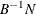### The Reduced Costs, Dual Activities, and Current Tableau

The evaluation of reduced costs and the dual activities is independent of problem structure. For a basic solution, let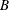be the matrix composed of the basic columns of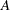and let
be the matrix composed of the nonbasic columns of. The reduced cost associated with theth variable is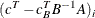and the dual activity of theth row is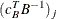The Current Tableau is a section displayed when you specify either the TABLEAUPRINT option in the PROC LP statement or the TABLEAU option in the PRINT statement. The output contains a row for each basic variable and a column for each nonbasic variable. In addition, there is a row for the reduced costs and a column for the product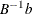This column is labeled `INV(B)*R`. The body of the tableau contains the matrix